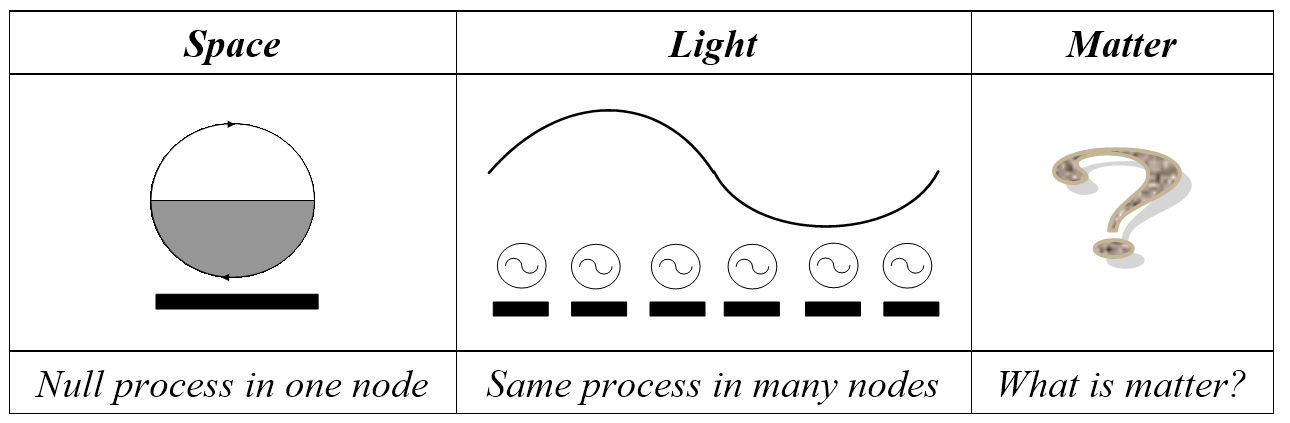# QR4.1 What is Matter?Figure 4.1. If a photon is space stretched out, what is matter?

Quantum realism explains space, time and light as follows:

·      Space. Space is a null quantum process running at a node point, so it is something that outputs “nothing”.

·      Time. Time is processing cycles completed, so if the network slows down, time can “dilate” as Einstein says.

·      Light. Light is one quantum process distributed over two or more nodes to give the electromagnetic spectrum.

If space is null processing, time is processing cycles completed and light is space distributed, can the same model explain matter? (Figure 4.1) If it can’t, the results so far are mere curiosities. Current physics explains matter by the standard model so a quantum model must explain all that it does and more.

Next